# Two best ways to convert numbers to words in Excel

In this article I will show you two quick and free ways to convert currency numbers into English words in Excel 2016, 2013 and other versions.

Microsoft Excel is a great program to calculate this and that. It was initially developed to process large data arrays. However, it also lets creating accounting records like invoices, evaluation or balance sheets quickly and effectively.

In more or less solid payment documents it is necessary to duplicate numeric values with their word form. It is much harder to falsify typed numbers than those written by hand. Some swindler can try to make 8000 out of 3000, while it is almost impossible to secretly replace "three" with "eight".

So what you need is not just convert numbers to words in Excel (е.g. 123.45 to "one hundred and twenty three, forty five"), but spell out dollars and cents (е.g. \$29.95 as "twenty nine dollars and ninety nine cents" ), pounds and pence for GBP, euros and eurocents for EUR, etc.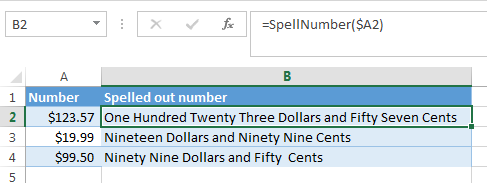Even Excel 2016 doesn't have a built-in tool for spelling numbers, not to mention earlier versions. But that is when Excel is really good. You can always improve its functionality using formulas in all their
combinations, VBA macros, or third-party add-ins.

Below you'll find two ways to convert numbers from figures to words

And, possibly, you may need to convert Words to Numbers in Excel

Note. If you are looking for the number to text conversion, which means you want Excel to see your number as text, it's a bit different thing. Usually, you simply need to change the cell format in Excel (select your range with numbers, press Ctrl+1 on the Number tab, and select "Text" in the Category field). You can also use the formula `=text()`.

Please find the details in the article Ways to convert numbers to text in Excel.

### SpellNumber VBA macro to convert numbers to words

As I have already mentioned, Microsoft didn't want to add a tool for this task. However, when they saw how many users needed it, they created and published the special VBA macro on their website. The macro does what its name SpellNumber suggests. All other macros I came across are based on the Microsoft code.

You can find the macro mentioned as "spellnumber formula". However, it is not a formula, but a macro function, or to be more precise Excel User defined function (UDF).

The spellnumber option is able to write dollars and cents. If you need a different currency, you can change "dollar" and "cent" with the name of your one.

If you are not a VBA savvy guy, below you will find a copy of the code. If you still don't want or haven't time to sort this out, please use this solution.

1. Open the workbook where you need to spell the numbers.
2. Press Alt+F11 to open the Visual Basic editor window.
3. If you have several books opened, check that the needed workbook is active using the list of projects in the upper left corner of the editor (one of the workbook elements is highlighted with blue).
4. In the editor menu go to Insert-> Module.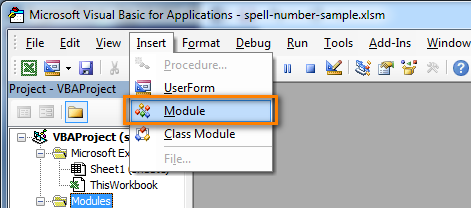5. You should see a window named YourBook - Module1. Select all of the code in the frame below and paste it to this window.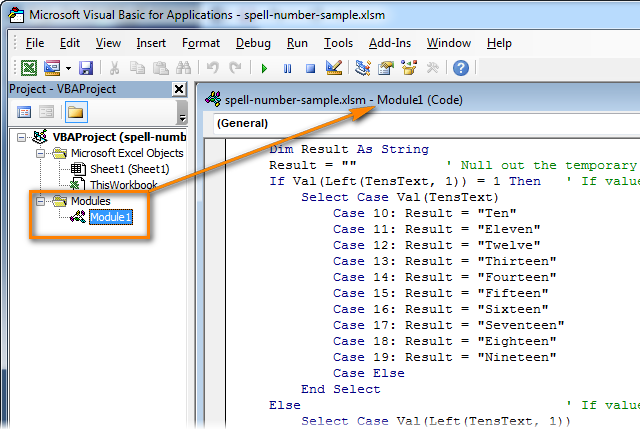```Option Explicit
'Main Function
Function SpellNumber(ByVal MyNumber)
Dim Dollars, Cents, Temp
Dim DecimalPlace, Count
ReDim Place(9) As String
Place(2) = " Thousand "
Place(3) = " Million "
Place(4) = " Billion "
Place(5) = " Trillion "

MyNumber = Trim(Str(MyNumber))
DecimalPlace = InStr(MyNumber, ".")
If DecimalPlace > 0 Then
Cents = GetTens(Left(Mid(MyNumber, DecimalPlace + 1) & _
"00", 2))
MyNumber = Trim(Left(MyNumber, DecimalPlace - 1))
End If
Count = 1
Do While MyNumber <> ""
Temp = GetHundreds(Right(MyNumber, 3))
If Temp <> "" Then Dollars = Temp & Place(Count) & Dollars
If Len(MyNumber) > 3 Then
MyNumber = Left(MyNumber, Len(MyNumber) - 3)
Else
MyNumber = ""
End If
Count = Count + 1
Loop
Select Case Dollars
Case ""
Dollars = "No Dollars"
Case "One"
Dollars = "One Dollar"
Case Else
Dollars = Dollars & " Dollars"
End Select
Select Case Cents
Case ""
Cents = " and No Cents"
Case "One"
Cents = " and One Cent"
Case Else
Cents = " and " & Cents & " Cents"
End Select
SpellNumber = Dollars & Cents
End Function

Function GetHundreds(ByVal MyNumber)
Dim Result As String
If Val(MyNumber) = 0 Then Exit Function
MyNumber = Right("000" & MyNumber, 3)
' Convert the hundreds place.
If Mid(MyNumber, 1, 1) <> "0" Then
Result = GetDigit(Mid(MyNumber, 1, 1)) & " Hundred "
End If
' Convert the tens and ones place.
If Mid(MyNumber, 2, 1) <> "0" Then
Result = Result & GetTens(Mid(MyNumber, 2))
Else
Result = Result & GetDigit(Mid(MyNumber, 3))
End If
GetHundreds = Result
End Function

Function GetTens(TensText)
Dim Result As String
Result = "" ' Null out the temporary function value.
If Val(Left(TensText, 1)) = 1 Then   ' If value between 10-19...
Select Case Val(TensText)
Case 10: Result = "Ten"
Case 11: Result = "Eleven"
Case 12: Result = "Twelve"
Case 13: Result = "Thirteen"
Case 14: Result = "Fourteen"
Case 15: Result = "Fifteen"
Case 16: Result = "Sixteen"
Case 17: Result = "Seventeen"
Case 18: Result = "Eighteen"
Case 19: Result = "Nineteen"
Case Else
End Select
Else ' If value between 20-99...
Select Case Val(Left(TensText, 1))
Case 2: Result = "Twenty "
Case 3: Result = "Thirty "
Case 4: Result = "Forty "
Case 5: Result = "Fifty "
Case 6: Result = "Sixty "
Case 7: Result = "Seventy "
Case 8: Result = "Eighty "
Case 9: Result = "Ninety "
Case Else
End Select
Result = Result & GetDigit _
(Right(TensText, 1))  ' Retrieve ones place.
End If
GetTens = Result
End Function

Function GetDigit(Digit)
Select Case Val(Digit)
Case 1: GetDigit = "One"
Case 2: GetDigit = "Two"
Case 3: GetDigit = "Three"
Case 4: GetDigit = "Four"
Case 5: GetDigit = "Five"
Case 6: GetDigit = "Six"
Case 7: GetDigit = "Seven"
Case 8: GetDigit = "Eight"
Case 9: GetDigit = "Nine"
Case Else: GetDigit = ""
End Select
End Function
```
6. Press Ctrl+S to save the updated workbook.

You will need to resave your workbook. When you try to save the workbook with a macro you'll get the message "The following features cannot be saved in macro-free workbook"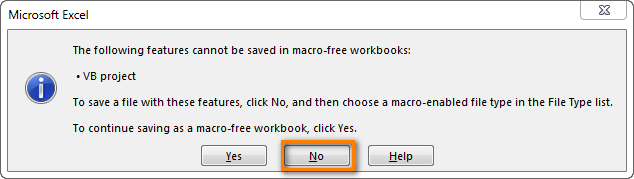Click No. When you see a new dialog, chose the Save as option. In the field "Save as type" pick the option "Excel macro-enabled workbook".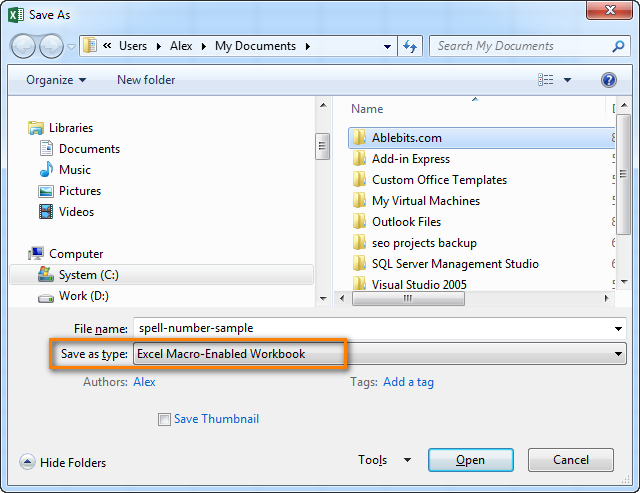#### Use SpellNumber macro in your worksheets

Now you can use the function SpellNumber in your Excel documents. Enter `=SpellNumber(A2)` into the cell where you need to get the number written in words. Here A2 is the address of the cell with the number or amount.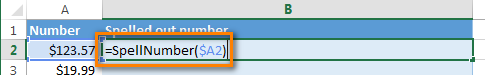Here you can see the result: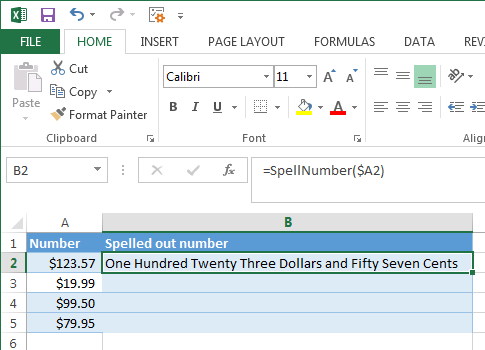Voila!

#### Quickly copy the SpellNumber function to other cells.

If you need to convert the entire table, not just 1 cell, place your mouse cursor to the lower right corner of the cell with the formula until it turns into a small black cross: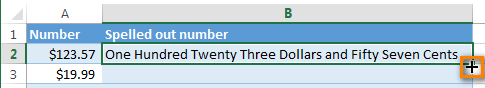Left-click and drag it across the column to fill in the formula. Release the button to see the results: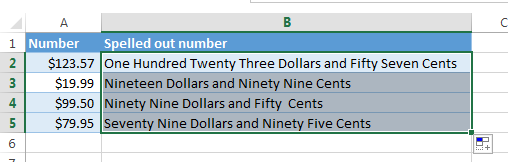Note. Please keep in mind that if you use SpellNumber with a link to another cell, the written sum will be updated each time the number in the source cell is changed.
You can also enter the number directly into the function, for example, `=SpellNumber(29.95)` (29.95 - without quotation marks and the Dollar sign).

#### Disadvantages of using macro to spell numbers in Excel

First off, you must know VBA to modify the code according to your needs. It is necessary to paste the code for each workbook, where you plan to change it. Otherwise, you will need to create a template file with macros and configure Excel to load this file at each start.

The main disadvantage of using a macro is if you send the workbook to somebody else, this person will not see the text unless the macro is built into the workbook. And even if it's built-in, they will get an alert that there are macros in the workbook.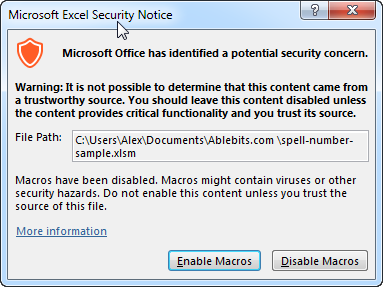For active Excel users who need to quickly spell sums but don't want to learn VBA or other workarounds, one kind developer created a free add-in - POPUP SPELL NUMBER for Microsoft Excel

Besides being ready for use, the tool is really flexible in converting numbers. You can choose the text case of the result (lower case, Title Case, Sentence case, UPPER CASE ), also you can select how you want to see the fraction:

• Spells the fraction in cent/cents, оr penny/pennies, оr penny/pence, or centavo/centavos
• Spells decimal point, each zero and the rest of the number as an integer.
• Doesn't spell the fraction but writes it as a fraction with denominator of 100, 1000, 1000000

The author states that it's tested and works in Excel for Windows 2000, 2002(XP), 2003, 2007, 2010 (32-bit), and 2013 (32-bit) in MSI-based and Click-To-Run installations of Office 365 cloud-based services.

#### And all is wonderful about POPUP SPELL NUMBER, but...

I hate to say that the add-in doesn't work with 64-bit versions of Excel 2010, 2013, 2007. And it is a considerable obstacle since these versions are becoming more and more popular. So lucky you are if you have Excel 32-bit :) Feel free to download the add-in and check it out.

If you are the one with Excel 64-bit like me, drop the author a line and ask him for a special version.

### Reverse conversion - English words into numbers

Frankly, I can't imagine why you may need it. Just in case... :)

It appears that Excel MVP, Jerry Latham, created such Excel User defined function (UDF) as WordsToDigits. It converts English words back to number.

You can download Jerry's WordsToDigits workbook to see the UDF code. Here you'll also find his examples of how to use the function.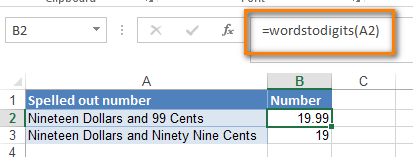You can see how the function works on the sheet "Sample Entries", where you will also be able to enter your own examples. If you plan to employ WordsToDigits in your documents, please be informed that this function has restrictions. For example, it doesn't recognize fractions entered in words. You will find all the details on the "Information" sheet.

### 351 Responses to "Two best ways to convert numbers to words in Excel"

1.Yohannes.melesse@syngenta .com says:

2.jeevan singh says:

Option Explicit
'Main Function
Function SpellNumber(ByVal MyNumber)
Dim Dollars, Cents, Temp
Dim DecimalPlace, Count
ReDim Place(9) As String
Place(2) = " Thousand "
Place(3) = " Million "
Place(4) = " Billion "
Place(5) = " Trillion "

MyNumber = Trim(Str(MyNumber))
DecimalPlace = InStr(MyNumber, ".")
If DecimalPlace > 0 Then
Cents = GetTens(Left(Mid(MyNumber, DecimalPlace + 1) & _
"00", 2))
MyNumber = Trim(Left(MyNumber, DecimalPlace - 1))
End If
Count = 1
Do While MyNumber ""
Temp = GetHundreds(Right(MyNumber, 3))
If Temp "" Then Dollars = Temp & Place(Count) & Dollars
If Len(MyNumber) > 3 Then
MyNumber = Left(MyNumber, Len(MyNumber) - 3)
Else
MyNumber = ""
End If
Count = Count + 1
Loop
Select Case Dollars
Case ""
Dollars = "No Dollars"
Case "One"
Dollars = "One Dollar"
Case Else
Dollars = Dollars & " Dollars"
End Select
Select Case Cents
Case ""
Cents = " and No Cents"
Case "One"
Cents = " and One Cent"
Case Else
Cents = " and " & Cents & " Cents"
End Select
SpellNumber = Dollars & Cents
End Function

Function GetHundreds(ByVal MyNumber)
Dim Result As String
If Val(MyNumber) = 0 Then Exit Function
MyNumber = Right("000" & MyNumber, 3)
' Convert the hundreds place.
If Mid(MyNumber, 1, 1) "0" Then
Result = GetDigit(Mid(MyNumber, 1, 1)) & " Hundred "
End If
' Convert the tens and ones place.
If Mid(MyNumber, 2, 1) "0" Then
Result = Result & GetTens(Mid(MyNumber, 2))
Else
Result = Result & GetDigit(Mid(MyNumber, 3))
End If
GetHundreds = Result
End Function

Function GetTens(TensText)
Dim Result As String
Result = "" ' Null out the temporary function value.
If Val(Left(TensText, 1)) = 1 Then ' If value between 10-19...
Select Case Val(TensText)
Case 10: Result = "Ten"
Case 11: Result = "Eleven"
Case 12: Result = "Twelve"
Case 13: Result = "Thirteen"
Case 14: Result = "Fourteen"
Case 15: Result = "Fifteen"
Case 16: Result = "Sixteen"
Case 17: Result = "Seventeen"
Case 18: Result = "Eighteen"
Case 19: Result = "Nineteen"
Case Else
End Select
Else ' If value between 20-99...
Select Case Val(Left(TensText, 1))
Case 2: Result = "Twenty "
Case 3: Result = "Thirty "
Case 4: Result = "Forty "
Case 5: Result = "Fifty "
Case 6: Result = "Sixty "
Case 7: Result = "Seventy "
Case 8: Result = "Eighty "
Case 9: Result = "Ninety "
Case Else
End Select
Result = Result & GetDigit _
(Right(TensText, 1)) ' Retrieve ones place.
End If
GetTens = Result
End Function

Function GetDigit(Digit)
Select Case Val(Digit)
Case 1: GetDigit = "One"
Case 2: GetDigit = "Two"
Case 3: GetDigit = "Three"
Case 4: GetDigit = "Four"
Case 5: GetDigit = "Five"
Case 6: GetDigit = "Six"
Case 7: GetDigit = "Seven"
Case 8: GetDigit = "Eight"
Case 9: GetDigit = "Nine"
Case Else: GetDigit = ""
End Select
End Function

•Loy says:

when i copy and paste this, it gives me errors, what am i doing wrong?

•Abhishek kumar says:

Option Explicit
'Main Function
Function SpellNumber(ByVal MyNumber)
Dim Rupees, Paisa, Temp
Dim DecimalPlace, Count
ReDim Place(9) As String
Place(2) = " Thousand "
Place(3) = " Million "
Place(4) = " Billion "
Place(5) = " Trillion "

MyNumber = Trim(Str(MyNumber))
DecimalPlace = InStr(MyNumber, ".")
If DecimalPlace > 0 Then
Paisa = GetTens(Left(Mid(MyNumber, DecimalPlace + 1) & _
"00", 2))
MyNumber = Trim(Left(MyNumber, DecimalPlace - 1))
End If
Count = 1
Do While MyNumber ""
Temp = GetHundreds(Right(MyNumber, 3))
If Temp "" Then Rupees = Temp & Place(Count) & Rupees
If Len(MyNumber) > 3 Then
MyNumber = Left(MyNumber, Len(MyNumber) - 3)
Else
MyNumber = ""
End If
Count = Count + 1
Loop
Select Case Rupees
Case ""
Rupees = "No Rupees"
Case "One"
Rupees = "One Rupees"
Case Else
Rupees = Rupees & " Rupees"
End Select
Select Case Paisa
Case ""
Paisa = " and No Paisa"
Case "One"
Paisa = " and One Paisa"
Case Else
Paisa = " and " & Paisa & " Paisa"
End Select
SpellNumber = Rupees & Paisa
End Function

Function GetHundreds(ByVal MyNumber)
Dim Result As String
If Val(MyNumber) = 0 Then Exit Function
MyNumber = Right("000" & MyNumber, 3)
' Convert the hundreds place.
If Mid(MyNumber, 1, 1) "0" Then
Result = GetDigit(Mid(MyNumber, 1, 1)) & " Hundred "
End If
' Convert the tens and ones place.
If Mid(MyNumber, 2, 1) "0" Then
Result = Result & GetTens(Mid(MyNumber, 2))
Else
Result = Result & GetDigit(Mid(MyNumber, 3))
End If
GetHundreds = Result
End Function

Function GetTens(TensText)
Dim Result As String
Result = "" ' Null out the temporary function value.
If Val(Left(TensText, 1)) = 1 Then ' If value between 10-19...
Select Case Val(TensText)
Case 10: Result = "Ten"
Case 11: Result = "Eleven"
Case 12: Result = "Twelve"
Case 13: Result = "Thirteen"
Case 14: Result = "Fourteen"
Case 15: Result = "Fifteen"
Case 16: Result = "Sixteen"
Case 17: Result = "Seventeen"
Case 18: Result = "Eighteen"
Case 19: Result = "Nineteen"
Case Else
End Select
Else ' If value between 20-99...
Select Case Val(Left(TensText, 1))
Case 2: Result = "Twenty "
Case 3: Result = "Thirty "
Case 4: Result = "Forty "
Case 5: Result = "Fifty "
Case 6: Result = "Sixty "
Case 7: Result = "Seventy "
Case 8: Result = "Eighty "
Case 9: Result = "Ninety "
Case Else
End Select
Result = Result & GetDigit _
(Right(TensText, 1)) ' Retrieve ones place.
End If
GetTens = Result
End Function

Function GetDigit(Digit)
Select Case Val(Digit)
Case 1: GetDigit = "One"
Case 2: GetDigit = "Two"
Case 3: GetDigit = "Three"
Case 4: GetDigit = "Four"
Case 5: GetDigit = "Five"
Case 6: GetDigit = "Six"
Case 7: GetDigit = "Seven"
Case 8: GetDigit = "Eight"
Case 9: GetDigit = "Nine"
Case Else: GetDigit = ""
End Select
End Function

•Abhishek kumar says:

than save ur file

•Dr. RAtnapal says:

i use this code but not given result. massage come Privacy warning; This documents contains macros, Activex controls,XML expansion pack information, or web components. These may include personal information that can not be removed by the documents inspector.

3.Marjorie Montemayor says:

how can i convert numbers to words for Peso

•Oli says:

replace lng mam yung dollars nang pesos (control+h) tapos replace all lng..

dollars to pesos
dollar to peso

4.Jorge Baez says:

I keep getting a syntax error
Do While MyNumber ""
Temp = GetHundreds(Right(MyNumber, 3))
If Temp "" Then Dollars = Temp & Place(Count) & Dollars

5.Supriya says:

Dear, this is to update you that the formula is not working perfectly. See the response that I am getting:->
For Kuwaiti Dinars
for KD 122.050 I got: One Hundred Twenty Two Kuwait Dinar and Five fils

the correct answer has to be: One Hundred Twenty Two Kuwait Dinar and Fifty fils
What to change now

•thon says:

look at the figure, it says .05 and not .50, the result is correct it seems the figure is point zero five, therefore the result should be five instead of fifty

•Eimantas says:

the issue is to replace the separator from "." to "," in the number or in the formula from:
MyNumber = Trim(Str(MyNumber))
DecimalPlace = InStr(MyNumber, ".")
to:
MyNumber = Trim(Str(MyNumber))
DecimalPlace = InStr(MyNumber, ",")

6.Supriya says:

I am getting a value like this :Four Hundred Eighty Dollars and Seventy One Cents. Instead of Dollars I want to have it in Kuwaiti Dinars and fills.
Pls help me

7.Tanzeel Hussain says:

I Was looking for him a lot, This formula hit is very good working on any Currency,
i am very happy thank you very much sir, Great job, May Allah bless you.😊😘💕😘💕😘💕😘💕

•Raja Zeeshan Khaliq says:

Assalam -o- Alaikum
My dear brother i want to convert a mathematical digits in english words without any currency like as
564 Five Hundred and Sixty Four
i have to prepare Result Cards

•Ikram Ullah says:

Wa Alaikum Assalam,
Dear Raja Zeeshan
Please in macro editor window press Ctrl+F , find Dollar and replace it with empty space . do it again for Dollars and similarly for Cent and cents respectively. I hope it will solve your problem.

8.Adil Farooqui says:

This formula is very good working on any Currency..

9.Liza says:

Thank you Sir for sharing this formula. It's help me a lot! I really appreciate that. Thanks again.

10.kherlen alih says:

help me naman po pano mag convert numbers to words sa excell for cheque please ..

11.Ola says:

Thank you for this.
However, this was noticed.
For example, i tried to spell 450,750.34
Four Hundred Fifty Thousand Seven Hundred Fifty Dollars and Thirty Four Cents
In my country, we spell like this
Four Hundred and Fifty Thousand, Seven Hundred and Fifty Dollars, Thirty Four Cents

•wasiullah says:

did u got that code? i need it too

•Bindesh says:

Option Explicit
'Main Function
Function SpellNumber(ByVal MyNumber)
Dim Rupees, Paisa, Temp
Dim DecimalPlace, Count
ReDim Place(9) As String
Place(2) = " Thousand "
Place(3) = " Million "
Place(4) = " Billion "
Place(5) = " Trillion "

MyNumber = Trim(Str(MyNumber))
DecimalPlace = InStr(MyNumber, ".")
If DecimalPlace > 0 Then
Paisa = GetTens(Left(Mid(MyNumber, DecimalPlace + 1) & _
"00", 2))
MyNumber = Trim(Left(MyNumber, DecimalPlace - 1))
End If
Count = 1
Do While MyNumber ""
Temp = GetHundreds(Right(MyNumber, 3))
If Temp "" Then Rupees = Temp & Place(Count) & Rupees
If Len(MyNumber) > 3 Then
MyNumber = Left(MyNumber, Len(MyNumber) - 3)
Else
MyNumber = ""
End If
Count = Count + 1
Loop
Select Case Rupees
Case ""
Rupees = "No Rupees"
Case "One"
Rupees = "One Dollar"
Case Else
Rupees = Rupees & " Rupees"
End Select
Select Case Paisa
Case ""
Paisa = " and No Paisa"
Case "One"
Paisa = " and One Cent"
Case Else
Paisa = " and " & Paisa & " Paisa"
End Select
SpellNumber = Rupees & Paisa
End Function

Function GetHundreds(ByVal MyNumber)
Dim Result As String
If Val(MyNumber) = 0 Then Exit Function
MyNumber = Right("000" & MyNumber, 3)
' Convert the hundreds place.
If Mid(MyNumber, 1, 1) "0" Then
Result = GetDigit(Mid(MyNumber, 1, 1)) & " Hundred "
End If
' Convert the tens and ones place.
If Mid(MyNumber, 2, 1) "0" Then
Result = Result & GetTens(Mid(MyNumber, 2))
Else
Result = Result & GetDigit(Mid(MyNumber, 3))
End If
GetHundreds = Result
End Function

Function GetTens(TensText)
Dim Result As String
Result = "" ' Null out the temporary function value.
If Val(Left(TensText, 1)) = 1 Then ' If value between 10-19...
Select Case Val(TensText)
Case 10: Result = "Ten"
Case 11: Result = "Eleven"
Case 12: Result = "Twelve"
Case 13: Result = "Thirteen"
Case 14: Result = "Fourteen"
Case 15: Result = "Fifteen"
Case 16: Result = "Sixteen"
Case 17: Result = "Seventeen"
Case 18: Result = "Eighteen"
Case 19: Result = "Nineteen"
Case Else
End Select
Else ' If value between 20-99...
Select Case Val(Left(TensText, 1))
Case 2: Result = "Twenty "
Case 3: Result = "Thirty "
Case 4: Result = "Forty "
Case 5: Result = "Fifty "
Case 6: Result = "Sixty "
Case 7: Result = "Seventy "
Case 8: Result = "Eighty "
Case 9: Result = "Ninety "
Case Else
End Select
Result = Result & GetDigit _
(Right(TensText, 1)) ' Retrieve ones place.
End If
GetTens = Result
End Function

Function GetDigit(Digit)
Select Case Val(Digit)
Case 1: GetDigit = "One"
Case 2: GetDigit = "Two"
Case 3: GetDigit = "Three"
Case 4: GetDigit = "Four"
Case 5: GetDigit = "Five"
Case 6: GetDigit = "Six"
Case 7: GetDigit = "Seven"
Case 8: GetDigit = "Eight"
Case 9: GetDigit = "Nine"
Case Else: GetDigit = ""
End Select
End Function

12.Sultan Ahmed says:

13.haider says:

i just want the wording not any currency. what should i do?

14.Guruprasad says:

Thank you Bro....

15.Jay says:

Hi, can i have the code without dollars and cents, E.g.
Four Million Six Hundred Three Thousand Four Hundred Twenty Two

16.Mujahid Azeem says:

Hi,
Could you tell me the code where I can mention "and" before the last figures e.g. Rs 1,987,290 (Rupees one million nine Hundred eighty seven thousand two hundred and ninety only)

17.Zainul Abdeen says:

Thanks you very much....

18.ARNON says:

SIMPLE, LOL, YOUR MACROS WORKED FIRST TIME, NO PROBLEMS, ONLY REQUEST IS FOR A CHANGE TO 00/00...01/00...03/00 AND SO ON INSTEAD OF CENTS, GOT YOURS TO WORK 00/00 AND 01/00 TO WORK, BUT AFTER THAT, ALL IS TEXT /00. SO I DELETED CENTS ALL TOGETHER TILL I GET THE CORRECT MACROS FOR THE OO/00 TO 99/00, PLEASE AND THANKS

•Arvin says:

I need the answer for this

19.Manjeet kimar says:

when i copy and paste this, it gives me errors, what am i doing wrong?

20.Mike says:

Hi, Can please I have the code without dollars and cents? thanks

•Raja Zeeshan Khaliq says:

Hey Mike!
do you get the code without dollars and cents
i also need this code
if you get this code please send me
i have really need this code

21.Yannick says:

It working very well. Thank's

22.Fahmid says:

Thanks for authentic codes. It works where codes from Microsoft gave an error message.
Best wushes!
Regards,

23.Marien says:

Thank you for this wonderful Module. Take care.

24.Lito Santos says:

tried it and it worked:
\$866.96 Eight Hundred Sixty Six Dollars and Ninety Five Cents
\$866.955 Eight Hundred Sixty Six Dollars and Ninety Five Cents
but it does not round up.
it is supposed to be :
Eight Hundred Sixty Six Dollars and Ninety Six Cents

•Lito Santos says:

please disregard the previous comment. the code is working fine.
i just need to use the roundup function in excel.

•Nik says:

You are a genius, thank you, I had the exact same problem.

25.Bobby Macias says:

Hello. I am wanted to enter the numbers 1 - 4 in each individual cell in an entire column. Once i insert the number and push ENTER, i want a text to replace the number based on which text i attach to that number. For example. The text for 1 is "Shut", i insert one into the cell and hit enter and it changes the value 1 to "shut

26.uzzal says:

thanks brother

27.Greg Lomboy says:

Why do I keep on getting #Name? Send Help please

•Bou Bou says:

me too

28.Govind says:

How To convert 48000 RS in English word in M S Excle

29.Houcine says:

Hi,
I need to convert a number with three digits after the decimal point, for example:
122,145 --> one hundred twenty two dollars one hundred forty five cents.

30.moo says:

Hi,I need the number without the code of dollers and cents,thanks

31.Hisham says:

Hello, the code is great! I'm using to print checks, so I need to add the word "only" at the end of the spelled number (ie twenty one dollars only). How can I achieve this?

32.Alvin says:

Hi,

I'd like to convert in this format
P151.50 = One Hundred Fifty-One & 50/100 only

Thanks,

•Alvin says:

UPDATE
I was able to solve this but works only on Windows 8, 8.1, & 10 Machines only
here is the code
Option Explicit

'****************' Main Function *'****************
Function SpellNumber(ByVal MyNumber)
Dim Pesos, Cents, Temp
Dim DecimalPlace, Count
ReDim Place(9) As String
Place(2) = " Thousand "
Place(3) = " Million "
Place(4) = " Billion "
Place(5) = " Trillion " ' String representation of amount
MyNumber = Trim(Str(MyNumber)) ' Position of decimal place 0 if none
DecimalPlace = InStr(MyNumber, ".")
'Convert cents and set MyNumber to Peso amount
If DecimalPlace > 0 Then
Cents = Right(MyNumber, 2)
MyNumber = Trim(Left(MyNumber, DecimalPlace - 1))
End If
Count = 1
Do While MyNumber ""
Temp = GetHundreds(Right(MyNumber, 3))
If Temp "" Then Pesos = Temp & Place(Count) & Pesos
If Len(MyNumber) > 3 Then
MyNumber = Left(MyNumber, Len(MyNumber) - 3)
Else
MyNumber = ""
End If
Count = Count + 1
Loop
Select Case Pesos
Case ""
Pesos = "No Pesos"
Case "One"
Pesos = "One Peso"
Case Else
Pesos = Pesos & " Pesos"
End Select
Select Case Cents
Case ""
Cents = " Only"
Case "1"
Cents = " & .1/100 Cent Only"
Case Else
Cents = " & " & (Cents) & "/100 Only"
End Select
SpellNumber = Pesos & Cents
End Function
'*******************************************
' Converts a number from 100-999 into text *
'*******************************************
Function GetHundreds(ByVal MyNumber)
Dim Result As String
If Val(MyNumber) = 0 Then Exit Function
MyNumber = Right("000" & MyNumber, 3) 'Convert the hundreds place
If Mid(MyNumber, 1, 1) "0" Then
Result = GetDigit(Mid(MyNumber, 1, 1)) & " Hundred "
End If
'Convert the tens and ones place
If Mid(MyNumber, 2, 1) "0" Then
Result = Result & GetTens(Mid(MyNumber, 2))
Else
Result = Result & GetDigit(Mid(MyNumber, 3))
End If
GetHundreds = Result
End Function
'*********************************************
' Converts a number from 10 to 99 into text. *
'*********************************************
Function GetTens(TensText)
Dim Result As String
Result = "" 'null out the temporary function value
If Val(Left(TensText, 1)) = 1 Then ' If value between 10-19
Select Case Val(TensText)
Case 10: Result = "Ten"
Case 11: Result = "Eleven"
Case 12: Result = "Twelve"
Case 13: Result = "Thirteen"
Case 14: Result = "Fourteen"
Case 15: Result = "Fifteen"
Case 16: Result = "Sixteen"
Case 17: Result = "Seventeen"
Case 18: Result = "Eighteen"
Case 19: Result = "Nineteen"
Case Else
End Select
Else ' If value between 20-99
Select Case Val(Left(TensText, 1))
Case 2: Result = "Twenty "
Case 3: Result = "Thirty "
Case 4: Result = "Forty "
Case 5: Result = "Fifty "
Case 6: Result = "Sixty "
Case 7: Result = "Seventy "
Case 8: Result = "Eighty "
Case 9: Result = "Ninety "
Case Else
End Select
Result = Result & GetDigit _
(Right(TensText, 1)) 'Retrieve ones place
End If
GetTens = Result
End Function
'*******************************************
' Converts a number from 1 to 9 into text. *
'*******************************************
Function GetDigit(Digit)
Select Case Val(Digit)
Case 1: GetDigit = "One"
Case 2: GetDigit = "Two"
Case 3: GetDigit = "Three"
Case 4: GetDigit = "Four"
Case 5: GetDigit = "Five"
Case 6: GetDigit = "Six"
Case 7: GetDigit = "Seven"
Case 8: GetDigit = "Eight"
Case 9: GetDigit = "Nine"
Case Else: GetDigit = ""
End Select
End Function

Sub test()
Dim number As Double
number = Range("A1").Value
Range("A2").Value = SpellNumber(number)
End Sub

33.Josef+ says:

Thanks a lot. i edited the currency bit from Dollars to GHCedis & Pesewas and it worked great! so grateful.

34.John Downes says:

Mate you are a genius - thanks a million

35.John Downes says:

Mate you are a genius - thanks a million

36.dan j says:

i got an error. "Ambiguous name detected: SpellNumber" ..how i gonna solve this?

Thanks a lot

37.Prashanth Nair says:

38.Umair Ahmad says:

done. Thank you so much

39.sakthivel says:

hai, i need to change the above coding to indian currency

40.SURAJMOHAN says:

hi guys
i cant use VBA in my company lap .
please help me to convert number to text in INR format only by suing any formulas without using macros

41.MITESH says:

Thanks a lot for sharing formula. Amazing...

42.saurav narvekar says:

dear sir,
what do if i want to spell a number in rupees ?
regard,
saurav narvekar

43.Likhith says:

It is very helpful for me. but some changes required.
if There is Multiple Decimals then how do we Convert calculation shows Wrong.
Example : 149.149 = Spellnumber(One Hundred Forty Nine Dollars and Fourteen Cents.
But it has to be fifteen Cents how to do it pls help.

44.Shah Faisal says:

Formula updated from Dollar to Rupees
Option Explicit
'Main Function
Function SpellNumber(ByVal MyNumber)
Dim Rupees, Paisa, Temp
Dim DecimalPlace, Count
ReDim Place(9) As String
Place(2) = " Thousand "
Place(3) = " Million "
Place(4) = " Billion "
Place(5) = " Trillion "

MyNumber = Trim(Str(MyNumber))
DecimalPlace = InStr(MyNumber, ".")
If DecimalPlace > 0 Then
Paisa = GetTens(Left(Mid(MyNumber, DecimalPlace + 1) & _
"00", 2))
MyNumber = Trim(Left(MyNumber, DecimalPlace - 1))
End If
Count = 1
Do While MyNumber ""
Temp = GetHundreds(Right(MyNumber, 3))
If Temp "" Then Rupees = Temp & Place(Count) & Rupees
If Len(MyNumber) > 3 Then
MyNumber = Left(MyNumber, Len(MyNumber) - 3)
Else
MyNumber = ""
End If
Count = Count + 1
Loop
Select Case Rupees
Case ""
Rupees = "No Rupees"
Case "One"
Rupees = "One Dollar"
Case Else
Rupees = Rupees & " Rupees"
End Select
Select Case Paisa
Case ""
Paisa = " and No Paisa"
Case "One"
Paisa = " and One Cent"
Case Else
Paisa = " and " & Paisa & " Paisa"
End Select
SpellNumber = Rupees & Paisa
End Function

Function GetHundreds(ByVal MyNumber)
Dim Result As String
If Val(MyNumber) = 0 Then Exit Function
MyNumber = Right("000" & MyNumber, 3)
' Convert the hundreds place.
If Mid(MyNumber, 1, 1) "0" Then
Result = GetDigit(Mid(MyNumber, 1, 1)) & " Hundred "
End If
' Convert the tens and ones place.
If Mid(MyNumber, 2, 1) "0" Then
Result = Result & GetTens(Mid(MyNumber, 2))
Else
Result = Result & GetDigit(Mid(MyNumber, 3))
End If
GetHundreds = Result
End Function

Function GetTens(TensText)
Dim Result As String
Result = "" ' Null out the temporary function value.
If Val(Left(TensText, 1)) = 1 Then ' If value between 10-19...
Select Case Val(TensText)
Case 10: Result = "Ten"
Case 11: Result = "Eleven"
Case 12: Result = "Twelve"
Case 13: Result = "Thirteen"
Case 14: Result = "Fourteen"
Case 15: Result = "Fifteen"
Case 16: Result = "Sixteen"
Case 17: Result = "Seventeen"
Case 18: Result = "Eighteen"
Case 19: Result = "Nineteen"
Case Else
End Select
Else ' If value between 20-99...
Select Case Val(Left(TensText, 1))
Case 2: Result = "Twenty "
Case 3: Result = "Thirty "
Case 4: Result = "Forty "
Case 5: Result = "Fifty "
Case 6: Result = "Sixty "
Case 7: Result = "Seventy "
Case 8: Result = "Eighty "
Case 9: Result = "Ninety "
Case Else
End Select
Result = Result & GetDigit _
(Right(TensText, 1)) ' Retrieve ones place.
End If
GetTens = Result
End Function

Function GetDigit(Digit)
Select Case Val(Digit)
Case 1: GetDigit = "One"
Case 2: GetDigit = "Two"
Case 3: GetDigit = "Three"
Case 4: GetDigit = "Four"
Case 5: GetDigit = "Five"
Case 6: GetDigit = "Six"
Case 7: GetDigit = "Seven"
Case 8: GetDigit = "Eight"
Case 9: GetDigit = "Nine"
Case Else: GetDigit = ""
End Select
End Function
-------------------------------
Regards

•Mihir Shah says:

Hey, thank you for the code. However, I am getting an error stating syntax error with Function SpellNumber(ByVal MyNumber) highlighted in yellow & Do while MyNumber"" highlighted too..

45.Bilal says:

Thanks. This works. But now the question is how to permanenelty add this formula in excel 2016 because I need this in many sheets and everytime i'm unable to add formula in excel.

46.jamshad says:

can i get code in uae dhirham and fils
in exel cell my total amount that amound i want to convert

47.akram says:

we require only mention in words, from any number for example
11,223,569 Eleven Million Two Hundred Twenty Three Thousand Five Hundred Sixty Nine Only
145,201 One Hundred Forty Five Thousand Two Hundred One Only
5,300 Five Thousand Three Hundred
Very Thanks
Akram Iqbal

48.Rajendra says:

This code is showing error for 100 crores it is showing 10 crores only

49.burhanuddin says:

can i change the currency like its dollars so can i change to dirhams and the fonts too

50.Rajesh says:

How to write formula for
45556.545
Forty Five Thousand Five Hundred Fifty six JOD and five hundred forty five fils

51.Glenn manlapaz says:

28,205.8

52.yousuf says:

I dont want to show in word in Paisa.
Now showing
4567.23= Taka Four Thousand Five hundred Sixty Seven twenty three paise only.
But I want to show Like this:
4567.23= Taka Four Thousand Five hundred Sixty Seven only.
Function SpellNumber(amt As Variant) As Variant
Dim FIGURE As Variant
Dim LENFIG As Integer
Dim i As Integer
Dim WORDs(19) As String
Dim tens(9) As String
WORDs(1) = "One"
WORDs(2) = "Two"
WORDs(3) = "Three"
WORDs(4) = "Four"
WORDs(5) = "Five"
WORDs(6) = "Six"
WORDs(7) = "Seven"
WORDs(8) = "Eight"
WORDs(9) = "Nine"
WORDs(10) = "Ten"
WORDs(11) = "Eleven "
WORDs(12) = "Twelve "
WORDs(13) = "Thirteen "
WORDs(14) = "Fourteen "
WORDs(15) = "Fifteen "
WORDs(16) = "Sixteen "
WORDs(17) = "Seventeen "
WORDs(18) = "Eighteen "
WORDs(19) = "Nineteen "
tens(2) = "Twenty "
tens(3) = "Thirty "
tens(4) = "Fourty "
tens(5) = "Fifty "
tens(6) = "Sixty "
tens(7) = "Seventy "
tens(8) = "Eighty "
tens(9) = "Ninety "
FIGURE = amt
FIGURE = Format(FIGURE, "FIXED")
FIGLEN = Len(FIGURE)
If FIGLEN 1 Then
SpellNumber = "Taka "
ElseIf Val(Left(FIGURE, 9)) = 1 Then
SpellNumber = "Taka "
End If
For i = 1 To 3
If Val(Left(FIGURE, 2)) 0 Then
SpellNumber = SpellNumber & WORDs(Val(Left(FIGURE, 2)))
ElseIf Val(Left(FIGURE, 2)) > 19 Then
SpellNumber = SpellNumber & tens(Val(Left(FIGURE, 1)))
SpellNumber = SpellNumber & WORDs(Val(Right(Left(FIGURE, 2), 1)))
End If
If i = 1 And Val(Left(FIGURE, 2)) > 0 Then
SpellNumber = SpellNumber & " Crore "
ElseIf i = 2 And Val(Left(FIGURE, 2)) > 0 Then
SpellNumber = SpellNumber & " Lakh "
ElseIf i = 3 And Val(Left(FIGURE, 2)) > 0 Then
SpellNumber = SpellNumber & " Thousand "
End If
FIGURE = Mid(FIGURE, 3)
Next i
If Val(Left(FIGURE, 1)) > 0 Then
SpellNumber = SpellNumber & WORDs(Val(Left(FIGURE, 1))) + " Hundred "
End If
FIGURE = Mid(FIGURE, 2)
If Val(Left(FIGURE, 2)) 0 Then
SpellNumber = SpellNumber & WORDs(Val(Left(FIGURE, 2)))
ElseIf Val(Left(FIGURE, 2)) > 19 Then
SpellNumber = SpellNumber & tens(Val(Left(FIGURE, 1)))
SpellNumber = SpellNumber & WORDs(Val(Right(Left(FIGURE, 2), 1)))
End If
FIGURE = Mid(FIGURE, 4)
If Val(FIGURE) > 0 Then
SpellNumber = SpellNumber & " Paise "
If Val(Left(FIGURE, 2)) 0 Then
SpellNumber = SpellNumber & WORDs(Val(Left(FIGURE, 2)))
ElseIf Val(Left(FIGURE, 2)) > 19 Then
SpellNumber = SpellNumber & tens(Val(Left(FIGURE, 1)))
SpellNumber = SpellNumber & WORDs(Val(Right(Left(FIGURE, 2), 1)))
End If
End If
FIGURE = amt
FIGURE = Format(FIGURE, "FIXED")
If Val(FIGURE) > 0 Then
SpellNumber = SpellNumber & " Only. "
End If
End Function

53.joel says:

I would like this one for Bahraini Dinar..as the whole process and change the currency there will be always an error. please help me on this...

54.Edge says:

Thank you for this. But how to do it like this: 190708 turn to (One-Nine-Zero-Seven-Zero-Eight)

Is there a way to do it like that? Thank you very much!

55.ALIDA says:

currently we are using spellnumber like this.
370,932.04 = Three Hundred Seventy Thousand Nine Hundred Thirty Two & 4/100 Pesos Only
But due to bank requirement it should be like this.
Three Hundred Seventy Thousand Nine Hundred Thirty Two & 04/100 Pesos Only

56.Nou Nang says:

Hi can anyone share me the excel add-in file of the Spell Number in USD? i cannot fine the excel Add-in File.

57.Jos says:

do you have the VBA code to convert numbers in French words

58.mark says:

can you remove the . before the cents and add a zero? eg. .1/100 Only to 10/100 Only

59.tusher says:

HOW TO MAKE IT APPLY ON EVERY EXCEL SHEET

60.Charles Afetorgbor says:

Convert
1.Four thousand three hundred and thirty to number figures
2.Three thousand and eighteen to number figures
3.Eight thousand and forty three to number figures
4.Nine thousand and seventy seven to number figures.
Convert
5. 9,44 to English words
6. 2,019 to English words
7. 7,003 to English words
Thanks.

61.Jepoy says:

Function SpellNumber(ByVal MyNumber) - -During running the formula , error occurs in MyNumber

62.Carol says:

Hi sir!
Good pm
Ask ko po sana pano po iconvert numbers to peso in excel 2016 windows 10.using this format po: ex.: 120.50
One Hundred Twenty Pesos and 50/100 Only
Thanks Sir! GOD BLESS

63.Shindie says:

what if i want to convert the numbers only without currency like "dollars" what will be the code?

64.ch venkataiah says:

how to convert marks in words up to 100 only.
65.Umer says: